# NCERT Solution Exercise 15.1 Probability Class 10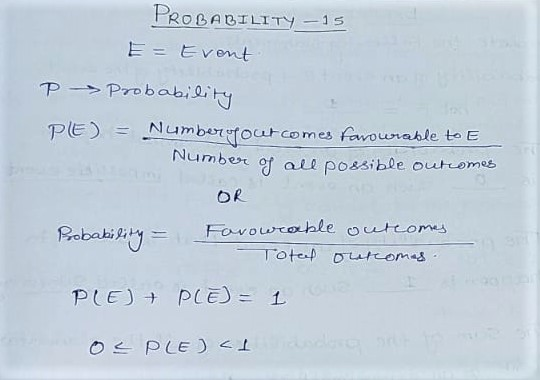Q1. Complete the Following Statements:

(i) Probability of an event E + Probability of the event ‘not E’ = 1

(ii) The Probability of an event that can not happen is  such an event is called an impossible event.

(iii) The probability of an event that is certain to happen issuch an event is called sure or a certain event.

(iv) The probability of an event is greater than or equal to and less than or equal to 1

Q3. Why is tossing a coin considered to be a fair way of deciding which team should get the ball at the beginning of a football game?

Ans: When we toss a coin the outcomes head and tail are equally likely .So, the result of an individual coin toss is completely unpredictable.

Q4. Which of the following cannot be the probability of an event ?

(A) 2/3

(B) -1.5

(C) 15%

(D) 0.7

Ans (B) 1.5 [Probability never be negative]

Q5. If P(E) = 0.05, what is the probability of ‘not E’?

Solution:

P(E) = 0.05

P(Ē) = not E =?

P(E) + P(Ē) = 1

P(Ē) = 1- P(E)

⇒ P(Ē) = 1- 0.05

⇒ P(Ē) = 0.95

Q6. A bag contains lemon-flavored candies only. Malini takes out one candy without looking into the bag. What is the probability that she takes out

(i) an orange-flavored candy?

(ii) a lemon-flavored candy?

Solution:

Probability of an orange-flavored candy = 0

Probability of a lemon-flavoured candy = 1

Q7. It is given that in a group of 3 students, the probability of 2 students not having the same birthday is 0.992. What is the probability that the 2 students have the same birthday?

Solution:

Let

P(Ē) = The probability of 2 students not having the same birthday = 0.992

P(E) = The probability of 2 students having the same birthday =?

We know that:

P(E) + P(Ē) = 1

⇒ P(E) = 1 – P(Ē)

⇒ P(E) = 1 – 0.992

⇒ P(E) = 0.008

Hence 2 students having the same birthday = 0.008

Q8. A bag contains 3 red balls and 5 black balls. A ball is drawn randomly from the bag. What is the probability that the ball drawn is (i) Red? (ii) not red?

Solution:

The total number of outcomes = Number of red balls + No.of black balls

⇒ The total number of outcomes = 3+5

⇒ The total number of outcomes = 8

(i) P(Red ball) = Favourable number of outcomes/Total number of outcomes

⇒ P(Red ball) = 3/8

(ii) P(Not red balls) = 1- P(red balls)

⇒ P(Not red balls) = 1- 3/8

⇒ P(Not red balls) = (8- 3)/8

⇒ P(Not red balls) = 5/8

Q9. A box Contains 5 red marbles, 8 white marbles, and 4 green marbles. What is the probability that the marble taken out will be:

(i) Red?

(ii) White?

(iii) not green?

Solution:

Number of Red marbles = 5

Number of White marbles = 8

Number of Green marbles = 4

Total number of marbles = 5+8+4 = 17

P(Red marbles) = Favourable number of outcomes/Total Number of outcomes

⇒ P(Red marbles) = 5/17

P(White marbles) = 8/17

P(not green marbles) =(Red + white)/17

⇒ P(not green marbles) =(5+8)/17

⇒ P(not green marbles) = 13/17

P(Red ball) = Favourable outcomes / Total number of outcomes

⇒ P(Red ball) = 13/17

Q 10. A Piggy bank contains hundred 50p coins, fifty Rs 1 coin, twenty Rs 2 coins and ten Rs 5 coins. If it is equally likely that one of the coins will fall out when the bank is turned upside down, What is the probability that the coin:

(i) Will be 50 p coins

(ii) Will not be a Rs 5 coin?

Solution:

Number of 50 Paisa coins = 100

Number of Rs 1 coins = 50

Number of Rs 2 coins = 20

Number of Rs 5 coins = 10

Total Number of coins = 180

(i) P(Will be 50 p coins) = Total number of 50 p coins/Total Number of coins

⇒ P(Will be 50 p coins) = 100/180

⇒ P(Will be 50 p coins) = 10/18

⇒ P(Will be 50 p coins) = 5/9

(ii) P(Will not be Rs 5 coin) = (Total Number of coins – number of Rs 5 coins)/Total number of coins

⇒ P(Will not be Rs 5 coin) = (180 – 10 )/180

⇒ P(Will not be Rs 5 coin) = 170/180

⇒ P(Will not be Rs 5 coin) = 17/18

Q 11. Gopi buys a fish From a shop for this aquarium. The Shopkeeper takes out one fish randomly From a tank containing 5 male fish and 8 female fish. What is the probability that the fish taken out is a male fish?

Solution:

Male Fish = 5

Female Fish = 8

Total Number of fish = 5+8 = 13

P(a male Fish) = Number of male Fish/Total Number of Fish

⇒ P(a male Fish) = 5/13

Q 12. A game of chance contains spinning an arrow that comes to rest pointing at one of the numbers 1,2,3,4,5,6,7,8 (see Fig. 15.5), and these are equally likely outcomes. What is the probability that it will point at?

(i)8

(ii) an odd number

(iii) a number greater than 2?

(iv) a number less than 9?

Solution:

Total Number of outcomes = 8

(i) P(8) = 1/8

(ii) an odd number = 1,3,5,7 = n(odd number) = 4

P(an odd number) = 4/8 = 1/2

(iii) A number greater than 2 = 3,4,5,6,7,8 = 6

P(a number greater than 2) = 6/8 = 3/4

(iv) a number less than 9 = 1,2,3,4,5,6,7,8 = 8

P(a number less than 9) = 8/8 =1

Q13. A die is thrown once. Find the probability of getting

(i) a prime number?

Solution:

Prime number = 2,3,5 = 3

P(a prime number) = 3/6 = 1/2

(ii) a number lying between 2 and 6 =?

Solution:

Number lying between 2 and 6 = 3,4,5 = 3

P(a number lying between 2 and 6 ) = 3/6 = 1/2

(iii) an odd number =?

Solution:

odd number =1,3,5 = 3

P(an odd number) = 3/6 = 1/2

Q14. One card is drawn from a well-shuffled deck of 52 cards. Find the probability of getting:

(i) a king of red color?

(ii) a face card?

(iii) a red face card?

(iv)The Jack of Hearts?

(v) The queen of diamonds?

Solution: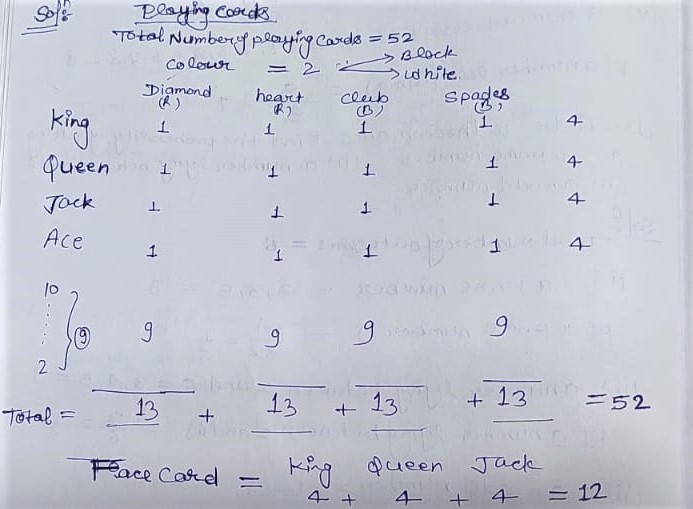Total number of playing cards = 52

(i) King of red color = 2

P(a king of red color) = Favourable outcomes/Total number of outcomes

⇒ P(a king of red color) = 2/52

⇒ P(a king of red color) = 1/26

(ii)

Number of face card = Number of King + Number of Queen + Number of jack = 4+4+4 = 12

P( a face card ) = 12/52 = 3/13

(iii)

Red Face card = King + Queen + Jack

⇒ Red Face card = 2 + 2 + 2 = 6

P(a Red Face card) = 6/52

⇒ P(a Red Face card) = 3/26

(iv) The jack of hearts = 1

P(the jack of hearts) = 1/26

(v) Number of spades = 13

P( a spade ) = 13/52

⇒ P( a spade ) = 1/4

(vi)

The Queen of Diamonds = 1

P(The queen of Diamonds) = 1/52

Q.15 Five cards- the ten, jack, queen, king, and ace of diamonds are well-shuffled with their face downwards.

one card is then picked up at random.

(i) What is the probability that the card is the queen?

(ii) If the queen is drawn and put aside, what is the probability that the second card picked up is:

(a) an ace?

(b) a queen?

Solution:

Five cards of diamonds= Ten + jack + queen + king + ace = 5

Total Number of Cards = 5

(i) P(the card is queen) = 1/5

(ii)

If the queen is drawn out and puts aside now Number of remaining total cards = 5 -1 = 4

(a) an ace = 1

P(an ace) = 1/4

(b) a queen = 1

P(a queen) = 0/4 = 0

Q16. 12 defective pens are accidentally mixed with 132 good ones. It is not possible to just look at a pen and tell whether or not it is defective. One pen is taken out at random from this lot. Determine the probability that the pen taken out is a good one.

Solution:

Number of Defective pens = 12

Number of Good Ones = 132

Total Number of Pen = 12+132 = 144

P(pen taken out is a good one) = Favourable outcomes/Total Number of outcomes

⇒ P(pen taken out is a good one) = 132/144

⇒ P(pen taken out is a good one) = 11/12

Q17. (i) A lot of 20 bulbs contain 4 defective ones. One bulb is drawn at random from the lot what is the probability that this bulb is defective?

(ii) Suppose the bulb drawn in (i) is not defective and is not replaced. Now one bulb is drawn at random from the rest. What is the probability that this bulb is not defective?

Solution:

(i) Total number of bulbs = 20

Defective bulbs = 4

Not defective bulbs = 20-4 = 16

P(The bulb defective) = Favourable outcomes / Total Number of outcomes

⇒ P(The bulb defective) = 4/20

⇒ P(The bulb defective) = 1/5

(ii) One bulb is drawn that is defective and Not Replaced then the Remaining total number of bulbs = 20-1=19

Number of not defective bulbs =16

P(Number of not defective bulbs) = 16/19

Q18. A box Contains 90 discs which are numbered from 1 to 90. If one disc is drawn at random from the box, Find the probability that it bears

(i) a two-digit number

(ii) a perfect square number

(iii) a number divisible by 5

Solution:

Total number of discs = 90

(i) Total Number of two digit Number = Total Number – One digit Number

⇒ Total Number of two digit Number = 90 – 9 = 81

P( a two-digit number) = 81/90

⇒ P( a two-digit number) = 9/10

P( a two-digit number) = 0.9

(ii) Perfect square number = 1,4,9,16,25,36,49,64,81,

Number of perfect square numbers = 9

P(a perfect square number) = 9/90

⇒ P(a perfect square number) = 1/10

⇒ P(a perfect square number) = 1/10 =0.1

(iii) Total number divisible by 5 between 1 to 90. = 5,10,15,20,25,30,35,40,45,50,55,60,65,70,75,80,85,90 = 18

P(a number divisible by 5) =18/90

⇒ P(a number divisible by 5) =1/5

Q19. A child has a die whose six faces show the letters as given below: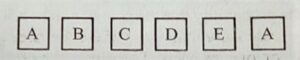the die is thrown one. What is the probability of getting (i) A? (ii) D?

Solution:

Total Number of outcomes = 6

(i) P(A) = 2/6 = 1/3

(ii) P(D) = 1/6

Q20. Suppose you drop a die at random on the rectangular region shown in Fig. 15.6. What is the probability that it will land inside the circle with a diameter of 1m?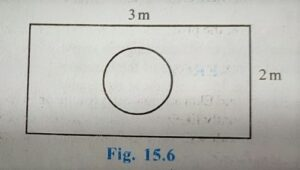Solution:

Total Number of outcomes =Area of rectangle

⇒ Total Number of outcomes = 3×2

⇒ Total Number of outcomes = 6 m

Favorable Number of outcomes = Area of circle

⇒ Favorable Number of outcomes = πr²

P(Die will land inside the circle) = πr²/6

⇒ P(Die will land inside the circle) = π(1/2)(1/2)/6

⇒ P(Die will land inside the circle) = π/2×2×6

⇒ P(Die will land inside the circle) = π/24

Q21. A lot consists of 144 ball pens of which 20 are defective and the others are good. Nuri will buy a pen if it is good, but will not buy it if it is defective. the Shopkeeper draws one pen at random and gives it to her. What is the probability that:

(ii) She will not buy it?

Solution:

Total number of ball pens = 144

Number of defective pens = 20

Number of good pens = 144-20 = 124

(i) P(She will buy it) = Number of good pens/Total number of pens

⇒ P(She will buy it) = 124/144

⇒ P(She will buy it) = 62/72

⇒ P(She will buy it) = 31/36

(ii) P(She will not buy it) = Number of defective pens/Total Number of pens

⇒ P(She will not buy it) = 20/144

⇒ P(She will not buy it) = 5/36

Q23. A Game consists of tossing a one rupee coin 3 times and noting its outcomes each time. Hanif eins if all the tosses give the same result. i.e., three heads or three tails, and loses otherwise. Calculate the probability that Hanif will lose the game.

Solution: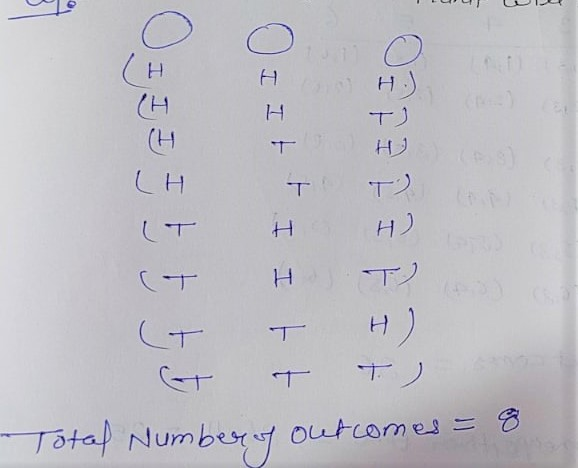Total Number of outcomes = 8

Favourable outcomes = If all three are same = (HHH),(TTT) = 2

P(Hanif wins) = 2/8 = 1/4

P(Hanif will lose the game) = 1-1/4

⇒ P(Hanif will lose the game) = (4-1)/4

P(Hanif will lose the game) = 3/4

or

P(Hanif will lose the game) =6/8 = 3/4

Q24. A die is thrown twice. What is the probability that

(i) 5 will not come up either time?

(ii) 5 will come up at least one?

Solution:

All possible outcomes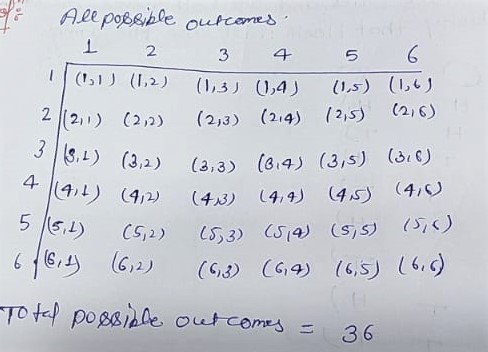Total possible outcomes = 36

(i) 5 will not come either time = 36 – 11 = 25

P(5 will not come up either time) = 25/36

(ii) 5 will come up at least one = 11

P(5 will come up at least one) = 11/36

Q25. Which of the following arguments are correct and which are not correct? Give reasons for your answer.

(i) If two coins are tossed simultaneously there are three possible outcomes – two heads two tails or one of each. Therefore, for each of these outcomes, the probability is 1/3

Solution:

When two coins are tossed, all possible outcomes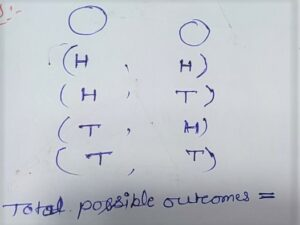S = { HH, HT, TH, TT}

P(HH) = 1/4

P( Two tail ) = 1/4

P(TT) = 1/4

P(one head or one tail ) = 2/4 = 1/2

∴ Given arguments are not true

(ii) If a die is thrown, there are two possible outcomes – an odd number or an even number. Therefore, the probability of getting an odd number is 1/2

Solution:

When a die is thrown once, total possible outcomes are: {1,2,3,4,5,6}

Number of possible outcomes = 6

Odd numbers = 1,2,5

∴ P(odd number) = 3/6=1/2

Even number = 2,4,6,

p(even number) = 3/6 = 1/2

Hence given argument is true.